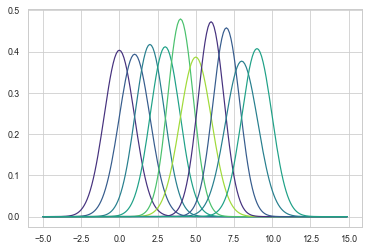# Understanding MultivariateNormal log_prob input semantics

Thanks to these two fantastic resources (I hope they help someone else), I understand how to instantiate batched Normal and MultivariateNormal objects:

I also understand the output shapes of sampling the batched distributions. However, I am somewhat lost as to the input shape requirements of the `log_prob` method.

Here’s an example implementation that I just got right through random permutation until it worked… I still have no idea how I did it:

``````# 1D mesh. Each point on the mesh will be the mean of a different distribution
mesh = torch.arange(0, 10)

scale = torch.ones_like(mesh, dtype=torch.float32)
scale += torch.normal(mean=0, std=torch.arange(0.1, 0.2, 0.01))

x_range = torch.arange(-5, 15, 0.1)
results = torch.exp(gaussians.log_prob(x_range))

for i, res in enumerate(results.T):
sns.lineplot(x=x_range, y=res)
``````

Okay, so I need to unsqueeze the input and it works fine…

``````results = torch.exp(gaussians.log_prob(x_range.unsqueeze(1)))
``````The 0th dimension is the number of samples. But what is this new dimension?

And what if I have a batch of samples:

``````x_range = torch.arange(-5, 15, 0.1)
x_range = torch.stack([x_range, x_range + 1]) # <--- Two batches

results = torch.exp(gaussians.log_prob(x_range))

for i, res in enumerate(results.T):
sns.lineplot(x=x_range, y=res)
``````

Again I get

So I unsqueeze the last dimension again. Now I’m dealing with three-dimensional input and I have no idea what two of the dimension are, nor the dimensions of the output.

Here is what the docs say:

Nothing…